# Knowledge of Power is Power

##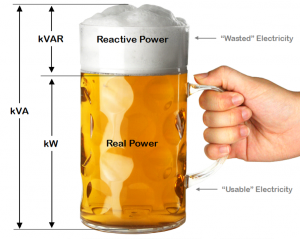A large beer is ordered to quench the thirst of a thirsty individual. The beer has some froth on top that does nothing to quench the individual’s thirst – this represents the kVAr (reactive power). The beer does quench the thirst – this represents the kW (real power). The total contents of the mug (the bear and the froth) represent the kVA or apparent power. The glass must be full of beer with no froth for the person to gain maximum benefit from the glass of beer. It is the same for maximum efficiency with power as the system should not be drawing any kVAr (or froth in the analogy).

What is Power? No, not the take-over-the-world kind of power. We’re talking about the kilowatts that power your life. Power is commonly talked about and used every single day, but what kind of power are you talking about and even more importantly, what kind of power are you using?

There are three types of power: Real Power, Reactive Power and Apparent Power. Real power is the type of power that is actually being used. It has units of Watts, though we normally see them as kilowatts [kW]. This power is what turns your lights on and your television on. Reactive power is power that is stored, but then released back into the source. It can be described as a “phantom power” because it looks like power is being used, but in reality there is no power loss. Reactive power has the units VAR for Volt-Ampere-Reactive [VAR]. Apparent power is the combination of real power and reactive power. Apparent power depicts the entire circuit’s total power and has the units Volt-Amperes [VA].

Are you confused yet? Let’s look at an analogy:
We have a horse pulling a cart that lays on a train track. The horse cannot stand on the train tracks so it must pull the cart on the right of the tracks. As the horse pulls the cart forward there are two forces acting on the cart: a force forward and a force perpendicular. The force forward is what helps move the cart across the tracks. The force perpendicular is rather useless, and takes up some of the horse’s effort. The combination of these two forces (or the hypotenuse in the picture) is the total amount of force acting on the cart.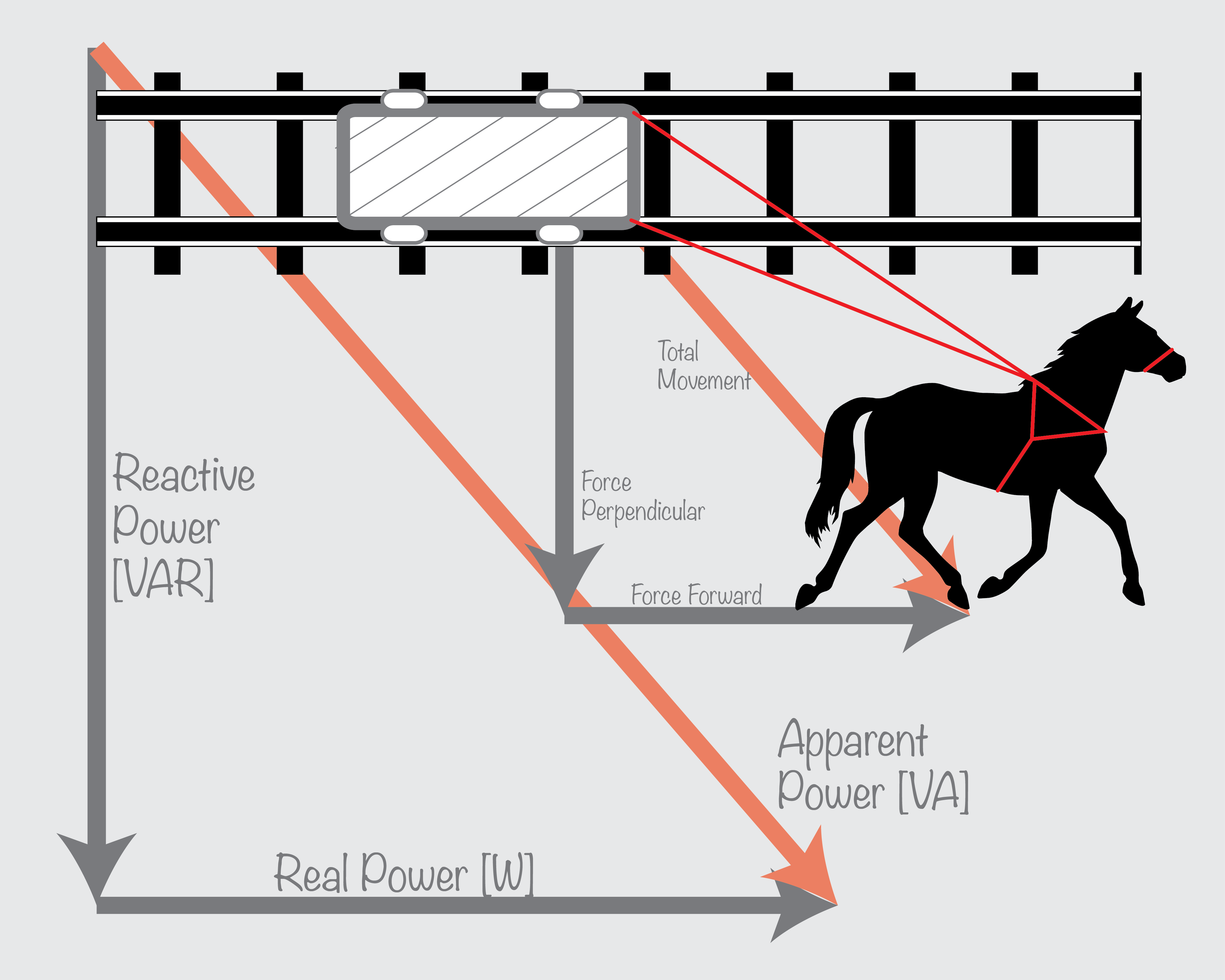The horse & cart analogy can be used to understand how real power, reactive power & apparent work.

We can apply the same idea of the forces to the different types of power. Real power is the equivalent of the forward force as it is what is used to power a device. Reactive power is similar to the perpendicular force as it exists but does not do any work. Of course, the total amount of force is similar to the apparent power for the total amount of power that is being used by a device.
Another helpful energy topic is power factor (also referred to as PF). Power factor is the ratio of the real power to the apparent power. In other words, power factor looks at the total amount of useful power to the total amount of power that is created in the circuit. This value can range from 0 to 1, with 1 being a perfect, or unity, power factor.
Let’s go back to the horse and cart analogy. The “power factor” of the horse is related to the amount of forward force the horse applies to the cart. As the horse moves closer to the tracks, the force perpendicular decreases and the power factor increases. The higher the power factor, the more motion or force that the horse can supply to move the cart forward.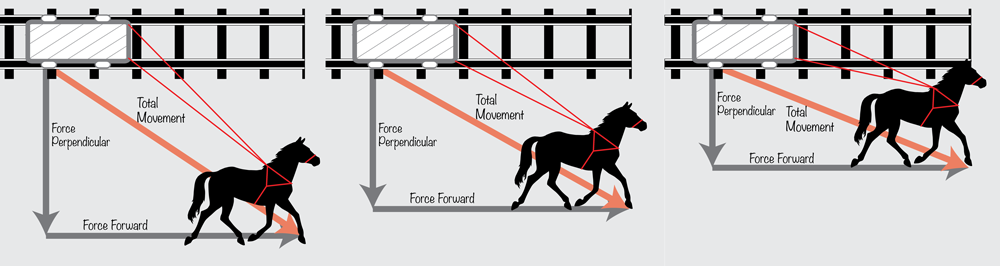As the horse rides closer to the tracks, the force producing no work decreases.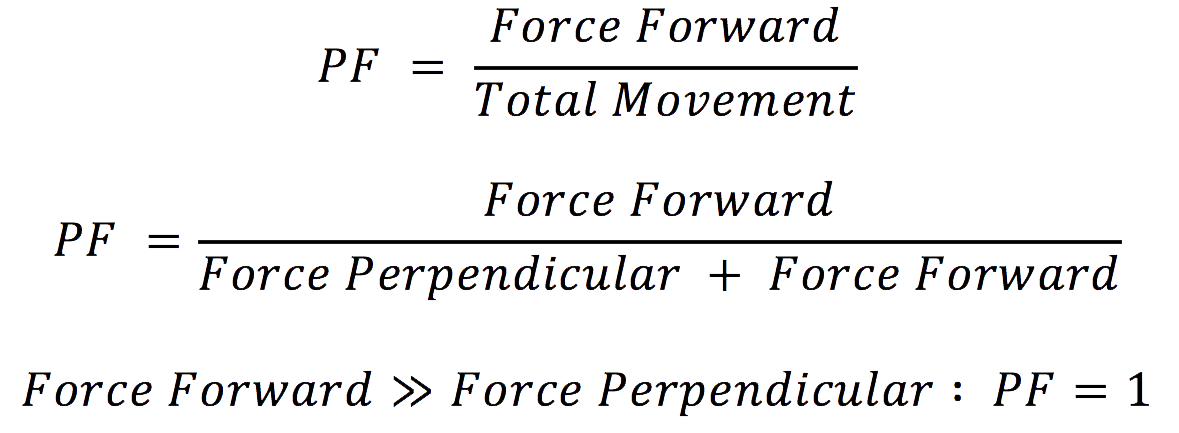We relate this back to the electrical power factor by looking at the how the entire circuit’s power is affected once the reactive power is decreased. The less reactive power available the more total power becomes real power.
Looking at the graphs below we compare real power to reactive power on a lower level. When the voltage and current running in a mechanism have very little to no phase difference (in other words, the two sine waves align), real power is created and is used by the circuit or load. You can see this from the AC voltage and AC current labeled on the graph. On the other hand, reactive power has current and voltage waves that are not aligned (90 degrees out of phase) so the summation of the waves create a net zero power output.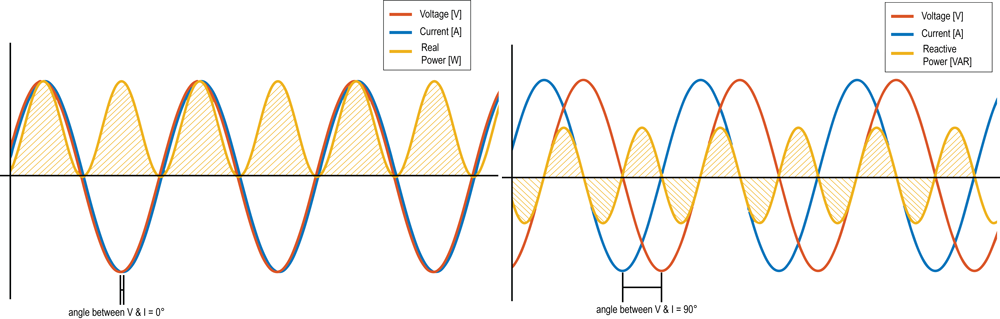Left: Real Power [W]; Right: Reactive Power [VAR]

So now the big question, why do you care about power and power factor?

Learning about the different types of power helps you understand your energy usage. HVAC systems, pumps and any type of induction motor produce a reactive power and a low power factor. This means there are higher inefficiencies in the power lines to your home or business and creates a higher demand for power. That high demand will cost you plenty as the utilities will penalize for anyone with a low factor. Corrections can be made using capacitive loads that will increase the power factor and help use less energy.

We use energy and power every day. It is important to know and understand how to use it most efficiently to keep sustainable methods and save money. Measure your power factor and understand where your energy is going.